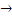## Monday, 27 April 2015

### SBI PO Reasoning Quiz -11

Directions (1 - 2) : Following questions are based on the five three digit numbers given below:

519 328 746 495 837

1. If half of the second highest number is subtracted from the third highest number, what will be the value ?

(1) 156 (2) 146 (3) 213 (4) 314 (5) None of these

2. If the positions of the first and the third digits in each of the numbers are interchanged, which of the following will be the second digit of the lowest number?

(1) 1 (2) 2 (3) 4 (4) 9 (5) 3

Direction (3 - 5): Study the following arrangement carefully and answer the questions given below :

H 9 3 P \$ K E % 4 F R 1 U @ W G 2 M 1 5 B 9 Z 6 © ⋆ N 6 8 V J

3. If all the symbols and numbers are dropped from the above arrangement, which of the following will be the fourteenth from the right end?

(1) M (2) K (3) W (4) E (5) None of these

4. What should come in place of the question mark (?) in the following series based on the above arrangement?

PK% RUW M5Q?

(1) ©N8 (2) ©N5 (3) 6⋆8 (4) 6⋆8 (5) None of these

5. How many such numbers are there in the above arrangement each of which is immediately preceded by a letter and immediately followed by a symbol ?

(1) None (2) One (3) Two (4) Three (5) More than three

Direction (6 - 10): In each of the questions below are given three statements followed by three conclusions numbered I, II and III. You have to take the given statements to be true even if they seem to be at variance from commonly known facts. Read all the conclusions and then decide which of the given conclusions logically follows from the given statements, disregarding commonly known facts.

6. Statements:

Some carrots are brinjals.

Some brinjals are apples.

All apples are bananas.

Conclusions:

I. Some apples are carrots.

II. Some bananas are brinjals.

III. Some bananas are carrots.

(1) Only I follows

(2) Only II follows

(3) Only III follows

(4) Only either II or III follows

(5) None of these

7. Statements:

All keys are locks.

All locks are bangles.

All bangles are cars.

Conclusions:

I. Some cars are locks.

II. Some bangles are keys.

III. Some cars are keys.

(1) Only I follows

(2) Only I and II follow

(3) Only I and III follow

(4) Only II and III follow

(5) All I, II and III follow

8. Statements:

All fruits are leaves.

Some leaves are trees.

No tree is house.

Conclusions:

I. Some houses are fruits.

II. Some trees are fruits.

III. No house is fruit.

(1) Only I follows

(2) Only II follows

(3) Only III follows

(4) Only either I or III follows

(5) None follows

9. Statements:

All tables are mirrors.

Some mirrors are chairs.

All chairs are glasses.

Conclusions:

I. Some glasses are mirrors,

II. Some chairs are tables.

III. Some mirrors are tables,

(1) Only I and II follow

(2) Only II and III follow

(3) Only I and III follow

(4) All I, II and III follow

(5) None of these

10. Statements:

All calculators are boxes.

All boxes are taps.

Some taps are machines.

Conclusions:

I. Some machines are boxes.

II. Some taps are calculators.

III. Some boxes are calculators.

(1) Only I and II follow

(2) Only I and III follow

(3) Only II and III follow

(4) All I, II and III follow

(5) None of these

1. 2; Second highest number

Third highest number = 519

Required difference

= 519 – 373 = 146

2. 4; 519 = 915: 328 = 823:

746 = 647: 495 = 594:

837 = 738

Lowest number = 594

3. 4; according to question, the new

Sequence would be :

4. 1;

5. 2;

 Letter Number Symbol

There is only one such combination : Z6©

(6 - 10):

Universal Affirmative (A-type)

Particular Affirmative (I-type).

(iii) No tree is houseUniversal Negative (E-type).

Particular Negative (O-type).

6. 2; Some brinjals are apples.

All apples are bananas.

I + A = I-type of Conclusions

Some brinjals are bananas.

Conclusions II is Converse of it.

7. 5; All keys are locks.

All locks are bangles.

A + A = A-type of Conclusions

All keys are bangles.

Conclusions II is Converse of it.

All locks are bangles.

All bangles are cars.

A + A = A-type of Conclusion

All locks are cars.

Conclusions I is Converse of it.

All keys are bangles.

All bangles are cars.

A + A = A-type of Conclusions

All keys are cars.

Conclusions III is Converse of it.

8. 4; Some leaves are trees.

No trees is house.

I + E = O-type of Conclusions

Some leaves are not houses.

Conclusions I and III form Complementary Pair.

Therefore, either I or III follows.

9. 3; Some mirrors are chairs.

All chairs are glasses.

I + A = I-type of Conclusions

Some mirrors are glasses.

Conclusions I is Converse of it.

Conclusion III is Converse of first premise.

10. 3; All calculators are boxes.

All boxes are taps.

A + A = A-type of Conclusion

All calculators are taps.

Conclusions II is Converse of it.

Conclusion III is Converse of first premise.